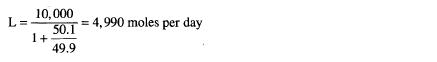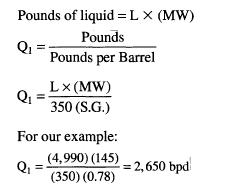﻿ Liquid Flow Rate | Oil & Gas Process Engineering

## Liquid Flow Rate

The liquid flow rate in moles per day for a given inlet stream can be determined from Equation 3-17. In our example, for an inlet stream rate of 10,000 moles per day, the liquid flow rate is:The liquid flow rate in barrels per day can be derived from:where Q1 = liquid flow rate, bpd
S.G. = specific gravity of liquid (water = 1)
Derivation of Equation 3-17
There are 350 pounds per barrel of water, and 350 (S.G.) pounds per barrel of liquid